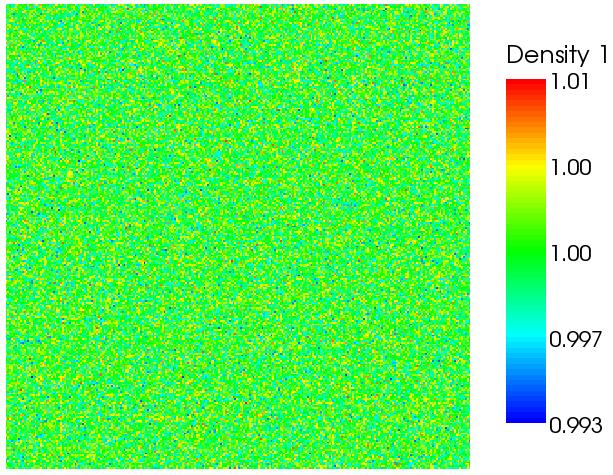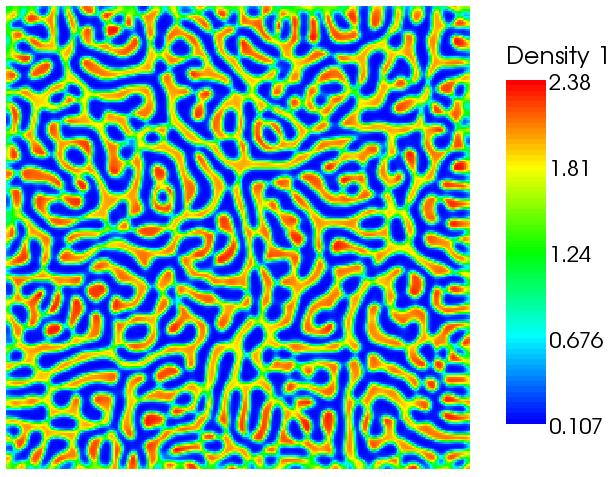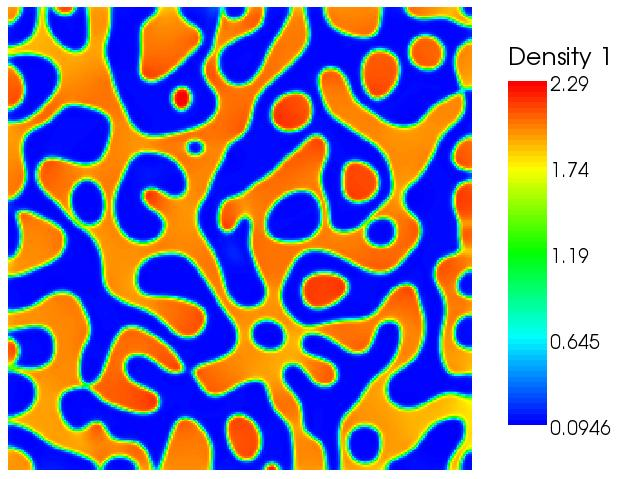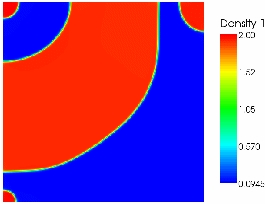# Implementation and validation of the multiphase Shan-Chen model in the Lattice Boltzmann Solver: waLBerla

Björn Gmeiner and Rishi Patil did their honours project at the Chair of System Simulation in Erlangen. waLBerla is a current research project at this chair.

Numerical simulations of multiphase flows are of engineering importance, due to the existence of multiphase flows in chemical and energy industries. Lattice Boltzmann Method (LBM) has emerged as an effective method for accurate simulations of fluid behavior. For multiphase simulations in LBM, the model developed by X. Shan and H. Chen is a standard model.

The aim of this project is to develop a Lattice Boltzmann solver for a wide range of applications. waLBerla is an acronym for widely applicable Lattice Boltzmann algorithm from Erlangen. The two phase model developed by Shan-Chen is implemented in waLBerla code. To verify the implementation, validation cases given in literature are tested.

Shan-Chen have described the model for 4d-hypercube model of LBM. Zhu, et al. have described the multiphase model for D3Q15 model which is similar to the D3Q19 model used in waLBerla. Hou. et al have done comparisons of Rothmann-Kellar (R-K) model and Shan-Chen models, and have described the validation cases used for comparison.

Two test cases have been used for validation. The first test case is a static bubble test, to test the stability of the implementation. Second test case is a dynamic case, which shows the separation of the phases from a initial random state. These test cases show that the Shan-Chen model has been successfully implemented in waLBerla. The capabilities and limitations of the implemented model and implementation are also investigated. Furthermore, the possible extensions are considered.The above figures show the separation of the two phases, starting from an initial random state.